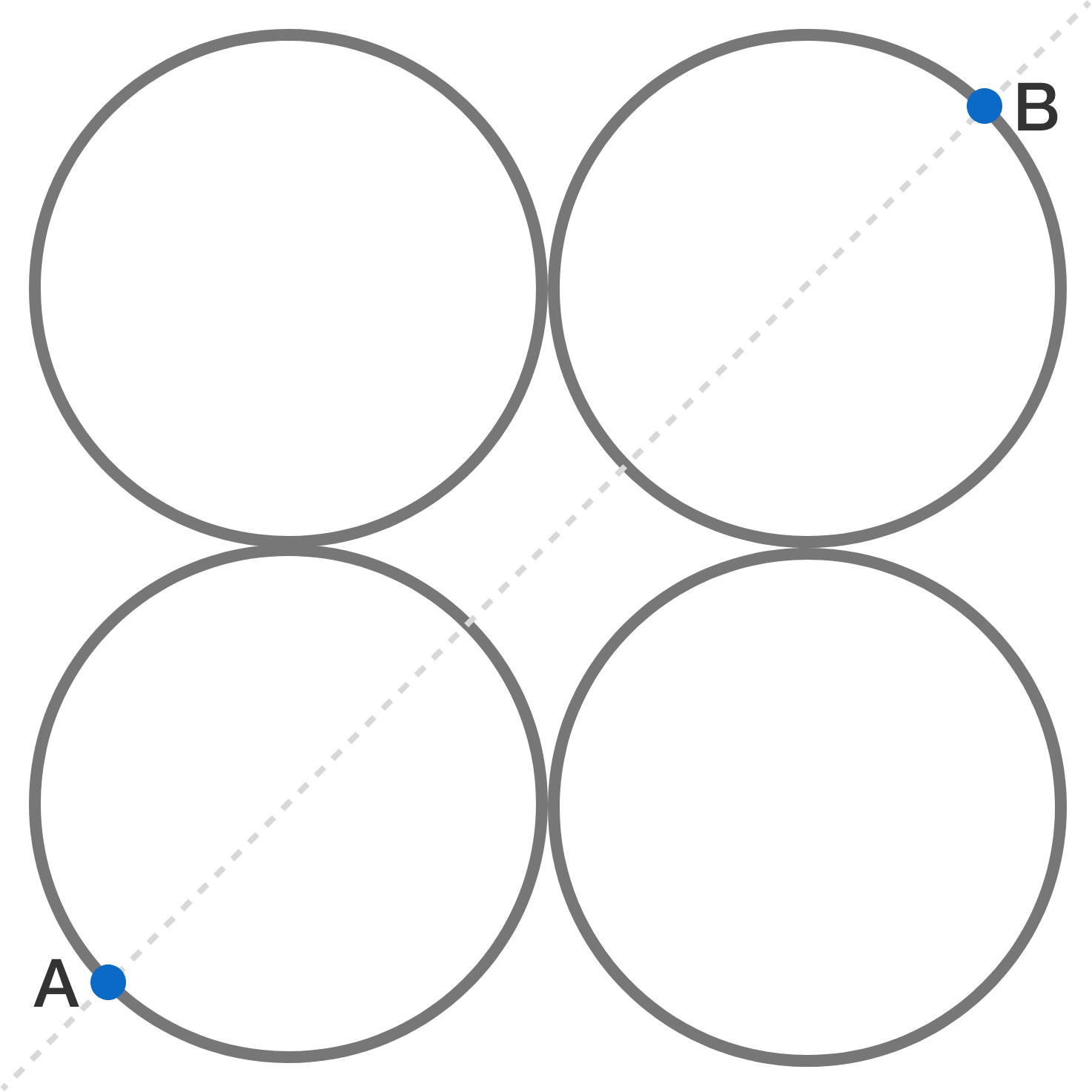# Resistance of Four RingsA uniform wire of resistance $R$ is cut into four circular rings of equal radius. Rings are then connected such that their centers lie on the vertices of a square (as illustrated in the figure above).

If the equivalent resistance between $A$ and $B$ can be expressed as $\frac{aR}{b}$, where $a$ and $b$ are coprime positive integers, determine the value of $a+b$.

×